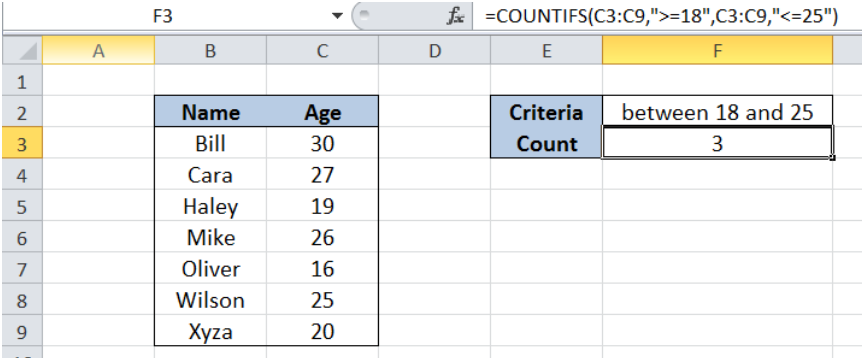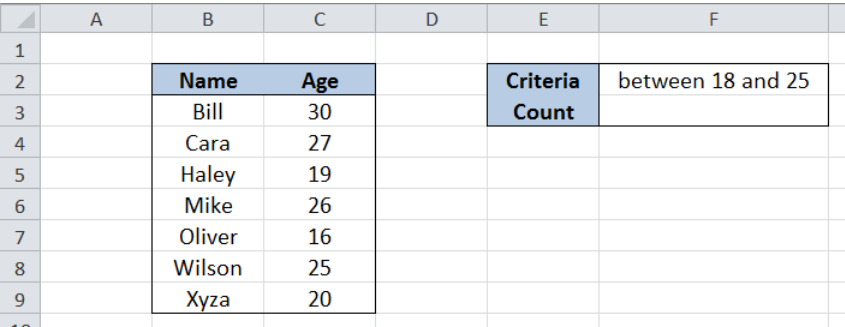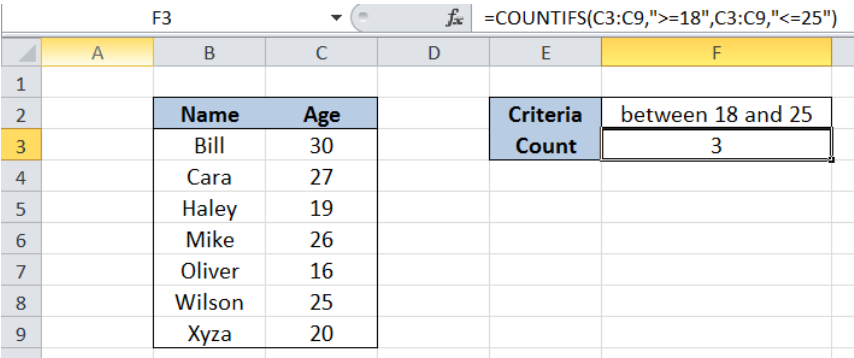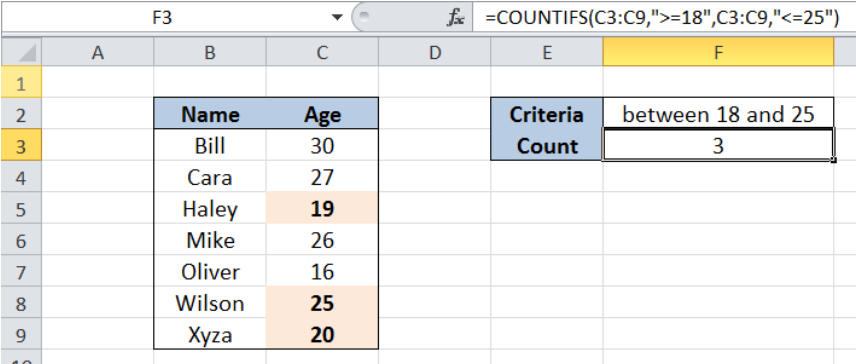Get instant live expert help with Excel or Google Sheets“My Excelchat expert helped me in less than 20 minutes, saving me what would have been 5 hours of work!”

#### Post your problem and you'll get expert help in seconds

Your message must be at least 40 characters
Our professional experts are available now. Your privacy is guaranteed.

# Count Cells Between Two Numbers

While working with Excel, we are able to count cells between two numbers by using the COUNTIFS function. The COUNTIFS function counts the number of cells that meet one or more criteria.  This step by step tutorial will assist all levels of Excel users in counting cells between two numbers.Figure 1. Final result: Count cells between two numbers

Final formula: `=COUNTIFS(C3:C9,">=18",C3:C9,"<=25")`

## Syntax of COUNTIFS Function

`=COUNTIFS(criteria_range1, criteria1, [criteria_range2, criteria2]…)`

Parameters:

• Criteria_range1: the data range that will be evaluated using the criteria1
• Criteria1:  the criteria or condition that determines which cells will be counted
• Criteria_range2 and criteria2 are optional; only applied when there are more than one criteria as specified

## Setting up Our Data

Our table contains a list of Names (column B) and Age (column C).  Cell F2 contains our criteria which is “between 18 and 25”. The resulting count will be in cell F3.Figure 2. Sample data to count cells between two numbers

## Count Cells Between Two Numbers

We want to determine the number of people in the list whose age falls between 18 and 25.

To count the number of cells between two numbers, we will follow these steps:

Step 1.  Select cell F3

Step 2.  Enter the formula: `=``COUNTIFS(C3:C9,">=18",C3:C9,"<=25")`

Step 3: Press ENTER

The range for our data set is C3:C9.  Our formula has two criteria.  It counts the cells in column C  with values between 18 and 25. The symbol “>=” means “greater than or equal to” while “<=” means “less than or equal to”.Figure 3. Using the COUNTIFS function to count cells between two numbers

The formula returns the value 3, which corresponds to the three cells with values between 18 and 25 as highlighted below.Figure 4. Highlighting the numbers between 18 and 25

Most of the time, the problem you will need to solve will be more complex than a simple application of a formula or function. If you want to save hours of research and frustration, try our live Excelchat service! Our Excel Experts are available 24/7 to answer any Excel question you may have. We guarantee a connection within 30 seconds and a customized solution within 20 minutes.

### Did this post not answer your question? Get a solution from connecting with the expert.Another blog reader asked this question today on Excelchat:## Subscribe to Excelchat.coAnother blog reader asked this question today on Excelchat: